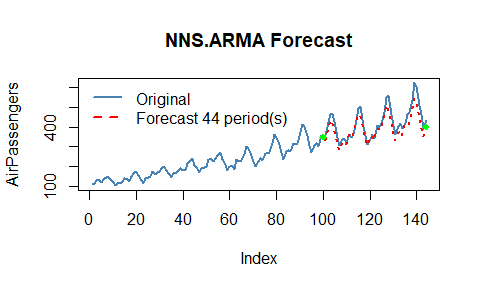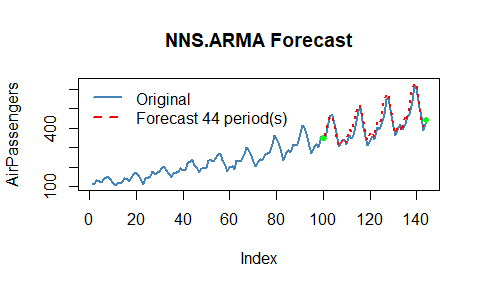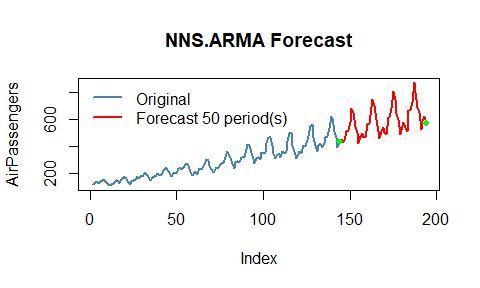# Getting Started with NNS: Forecasting

require(NNS)
require(knitr)
require(rgl)
require(data.table)
require(dtw)

# Forecasting

The underlying assumptions of traditional autoregressive models are well known. The resulting complexity with these models leads to observations such as,

We have found that choosing the wrong model or parameters can often yield poor results, and it is unlikely that even experienced analysts can choose the correct model and parameters efficiently given this array of choices.’’ Source

NNS simplifies the forecasting process. Below are some examples demonstrating NNS.ARMA and its assumption free, minimal parameter forecasting method.

## Linear Regression

NNS.ARMA has the ability to fit a linear regression to the relevant component series, yielding very fast results. For our running example we will use the AirPassengers dataset loaded in base R.

We will forecast 44 periods h = 44 of AirPassengers using the first 100 observations training.set = 100, returning estimates of the final 44 observations. We will then test this against our validation set of tail(AirPassengers,44).

Since this is monthly data, we will try a seasonal.factor = 12.

Below is the linear fit and associated root mean squared error (RMSE) using method = "lin".

nns = NNS.ARMA(AirPassengers, h = 44, training.set = 100, method = "lin", plot = TRUE, seasonal.factor = 12, seasonal.plot = FALSE, ncores = 1)sqrt(mean((nns - tail(AirPassengers, 44)) ^ 2))
##  35.39965

## Nonlinear Regression

Now we can try using a nonlinear regression on the relevant component series using method = "nonlin".

nns = NNS.ARMA(AirPassengers, h = 44, training.set = 100, method = "nonlin", plot = FALSE, seasonal.factor = 12, seasonal.plot = FALSE, ncores = 1)

sqrt(mean((nns - tail(AirPassengers, 44)) ^ 2))
##  27.0248

## Cross-Validation

We can test a series of seasonal.factors and select the best one to fit. The largest period to consider would be 0.5 * length(variable), since we need more than 2 points for a regression! Remember, we are testing the first 100 observations of AirPassengers, not the full 144 observations.

seas = t(sapply(1 : 25, function(i) c(i, sqrt( mean( (NNS.ARMA(AirPassengers, h = 44, training.set = 100, method = "lin", seasonal.factor = i, plot=FALSE, ncores = 1) - tail(AirPassengers, 44)) ^ 2) ) ) ) )

colnames(seas) = c("Period", "RMSE")
seas
##       Period      RMSE
##  [1,]      1  75.67783
##  [2,]      2  75.71250
##  [3,]      3  75.87604
##  [4,]      4  75.16563
##  [5,]      5  76.07418
##  [6,]      6  70.43185
##  [7,]      7  77.98493
##  [8,]      8  75.48997
##  [9,]      9  79.16378
## [10,]     10  81.47260
## [11,]     11 106.56886
## [12,]     12  35.39965
## [13,]     13  90.98265
## [14,]     14  95.64979
## [15,]     15  82.05345
## [16,]     16  74.63052
## [17,]     17  87.54036
## [18,]     18  74.90881
## [19,]     19  96.96011
## [20,]     20  88.75015
## [21,]     21 100.21346
## [22,]     22 108.68674
## [23,]     23  85.06430
## [24,]     24  35.49018
## [25,]     25  75.16192

Now we know seasonal.factor = 12 is our best fit, we can see if there’s any benefit from using a nonlinear regression. Alternatively, we can define our best fit as the corresponding seas$Period entry of the minimum value in our seas$RMSE column.

a = seas[which.min(seas[ , 2]), 1]

Below you will notice the use of seasonal.factor = a generates the same output.

nns = NNS.ARMA(AirPassengers, h = 44, training.set = 100, method = "nonlin", seasonal.factor = a, plot = TRUE, seasonal.plot = FALSE, ncores = 1)sqrt(mean((nns - tail(AirPassengers, 44)) ^ 2))
##  27.0248

Note: You may experience instances with monthly data that report seasonal.factor close to multiples of 3, 4, 6 or 12. For instance, if the reported seasonal.factor = {37, 47, 71, 73} use (seasonal.factor = c(36, 48, 72)) by setting the modulo parameter in NNS.seas(..., modulo = 12). The same suggestion holds for daily data and multiples of 7, or any other time series with logically inferred cyclical patterns. The nearest periods to that modulo will be in the expanded output.

NNS.seas(AirPassengers, modulo = 12, plot = FALSE)
## $all.periods ## Period Coefficient.of.Variation Variable.Coefficient.of.Variation ## 1: 48 0.4002249 0.4279947 ## 2: 12 0.4059923 0.4279947 ## 3: 60 0.4279947 0.4279947 ## 4: 36 0.4279947 0.4279947 ## 5: 24 0.4279947 0.4279947 ## 6: 1 0.4279947 0.4279947 ## ##$best.period
##  48
##
## $periods ##  48 12 60 36 24 1 ## Cross-Validating All Combinations of seasonal.factor NNS also offers a wrapper function NNS.ARMA.optim() to test a given vector of seasonal.factor and returns the lowest objective function (in this case RMSE written as expression()) and the optimal periods, as well as the NNS.ARMA regression method used. Given our monthly dataset, we will try multiple years by setting seasonal.factor = seq(12, 24, 6) every 6 months. nns.optimal = NNS.ARMA.optim(AirPassengers[1:100], training.set = 88, seasonal.factor = seq(12, 24, 6), obj.fn = expression( sqrt(mean((predicted - actual)^2)) ), ncores = 1) ##  "CURRNET METHOD: lin" ##  "COPY LATEST PARAMETERS DIRECTLY FOR NNS.ARMA() IF ERROR:" ##  "NNS.ARMA(... method = 'lin' , seasonal.factor = c( 12 ) ...)" ##  "CURRENT lin OBJECTIVE FUNCTION = 27.0536971551377" ##  "BEST method = 'lin', seasonal.factor = c( 12)" ##  "BEST lin OBJECTIVE FUNCTION = 27.0536971551377" ##  "CURRNET METHOD: nonlin" ##  "COPY LATEST PARAMETERS DIRECTLY FOR NNS.ARMA() IF ERROR:" ##  "NNS.ARMA(... method = 'nonlin' , seasonal.factor = c( 12 ) ...)" ##  "CURRENT nonlin OBJECTIVE FUNCTION = 13.9204086785474" ##  "BEST method = 'nonlin' PATH MEMBER = c( 12)" ##  "BEST nonlin OBJECTIVE FUNCTION = 13.9204086785474" ##  "CURRNET METHOD: both" ##  "COPY LATEST PARAMETERS DIRECTLY FOR NNS.ARMA() IF ERROR:" ##  "NNS.ARMA(... method = 'both' , seasonal.factor = c( 12 ) ...)" ##  "CURRENT both OBJECTIVE FUNCTION = 17.9477979123009" ##  "BEST method = 'both' PATH MEMBER = c( 12)" ##  "BEST both OBJECTIVE FUNCTION = 17.9477979123009" nns.optimal ##$periods
##  12
##
## $weights ## NULL ## ##$obj.fn
##  13.92041
##
## $method ##  "nonlin" ## ##$bias.shift
##  0

Using our new parameters yields the same results:

nns = NNS.ARMA(AirPassengers, training.set = 100, h = 44, seasonal.factor = nns.optimal$periods, weights = nns.optimal$weights, method = nns.optimal$method, plot = TRUE, seasonal.plot = FALSE, ncores = 1)sqrt(mean((nns - tail(AirPassengers, 44)) ^ 2)) ##  27.0248 ## $bias.shift

NNS.ARMA.optim will return a $bias.shift, which is to be added to the ultimate NNS.ARMA forecast when using the optimum parameters from the NNS.ARMA.optim call. sqrt(mean((nns+nns.optimal$bias.shift - tail(AirPassengers, 44)) ^ 2))
##  27.0248

### Negative values and $bias.shift If the variable cannot logically assume negative values, then simply limit the NNS estimates. nns <- pmax(0, nns+nns.optimal$bias.shift)
sqrt(mean((nns - tail(AirPassengers, 44)) ^ 2))
##  27.0248

## Extension of Estimates

Using our cross-validated parameters (seasonal.factor and method) we can forecast another 50 periods out-of-range (h = 50), by dropping the training.set parameter.

NNS.ARMA(AirPassengers, h = 50, seasonal.factor = nns.optimal$periods, method = nns.optimal$method, weights = nns.optimal$weights, plot = TRUE, seasonal.plot = FALSE, ncores = 1) + nns.optimal$bias.shift## Brief Notes on Other Parameters

• seasonal.factor = c(1, 2, ...)

We included the ability to use any number of specified seasonal periods simultaneously, weighted by their strength of seasonality. Computationally expensive when used with nonlinear regressions and large numbers of relevant periods.

• weights

Instead of weighting by the seasonal.factor strength of seasonality, we offer the ability to weight each per any defined compatible vector summing to 1.
Equal weighting would be weights = rep((1/length(seasonal.factor)), length(seasonal.factor)).

• seasonal.factor = FALSE

We also included the ability to use all detected seasonal periods simultaneously, weighted by their strength of seasonality. Computationally expensive when used with nonlinear regressions and large numbers of relevant periods.

• best.periods

This parameter restricts the number of detected seasonal periods to use, again, weighted by their strength. To be used in conjunction with seasonal.factor = FALSE.

• dynamic = TRUE

This setting generates a new seasonal period(s) using the estimated values as continuations of the variable, either with or without a training.set. Also computationally expensive due to the recalculation of seasonal periods for each estimated value.

• plot , seasonal.plot and intervals

These are the plotting arguments, easily enabled or disabled with TRUE or FALSE. seasonal.plot = TRUE will not plot without plot = TRUE. If a seasonal analysis is all that is desired, NNS.seas is the function specifically suited for that task. intervals will plot the surrounding estimated values iff intervals = TRUE & seasonal.factor = FALSE.

# Multivariate Time Series Forecasting

The extension to a generalized multivariate instance is provided in the following documentation of the NNS.VAR() function: